# GSEB Solutions Class 8 Maths Chapter 3 Understanding Quadrilaterals Ex 3.3

Gujarat Board GSEB Textbook Solutions Class 8 Maths Chapter 3 Understanding Quadrilaterals Ex 3.3 Textbook Questions and Answers.

## Gujarat Board Textbook Solutions Class 8 Maths Chapter 3 Understanding Quadrilaterals Ex 3.3

Question 1.
Given a parallelogram ABCD. Complete each statement along with the definition or property used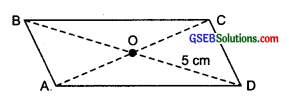(ii) ZDCB = __________
(in) OC = __________
(iv) ∠DAB + mZCDA = __________
Solution:
(i) AD = BC [v Opposite sides are equal]
(ii) ZDCB = ZDAB [ Opposite angles are equal]
(iii) OC = OA [Y Diagonals bisect each other]
(iv) mZDAB + mZCDA = 180°Question 2.
Consider the following parallelograms. Find the values of the unknowns x, y, z.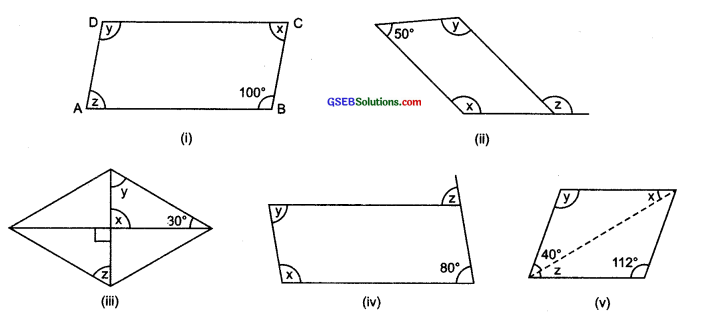Solution:
(i) ∠y = 100°
[∴ Opposite angles of a parallelogram are equal.]
Sum of interior angles of a parallelogram = 360°
∴ x + y + z + ∠B = 360°
or x + 100° + z + 100° = 360°
or x + z = 360° – 100° – 100° = 160°
But x = z
∴ x = z = $$\frac { 160° }{ 2 }$$ = 80°
Thus,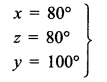(ii) ∴ Opposite angles are equal.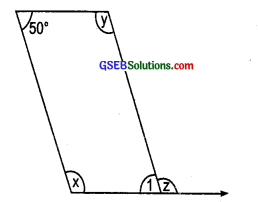∴ m ∠1 = 50
now, ∠1 + z = 180° [Linear pair]
or z = 180° – ∠1
= 180° – 50° = 130°
x + y + 50° + 50° = 360°
or x + y = 360° – 50° – 50° = 260°
But x = y
∴ x = y = $$\frac { 260 }{ 2 }$$ = 130°
Thus,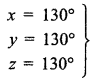(iii) ∵Vertically opposite angles are equal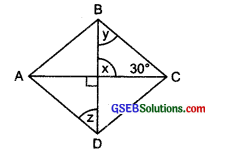∵ x = 90°
∵ Sum of the angles of a triangle = 180°
∴ 90° + 30° + y = 180°
or y = 108° – 30° – 90° = 60°
In the figure ABCD is a parallelogram
∴ AD || BC and BD is a transversal
∴ y = z [Alternate angles]
But y = 60°
∴ z = 60°
Thus, x = 90°, y = 60° and z = 60°(iv) ABCD is a parallelogram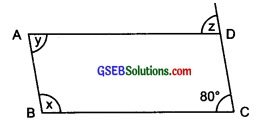∴ Opposite angles are equal.
∴ y = 80°
AB || CD and BC is a transversal.
∴ x + 80° = 180°
[Interior opposite angles]
or x = 180° – 80° = 100°
Again BC || AD and CD is a transversal,
∴ z = 80° [Corresponding angles]
Thus, x = 100°, y = 80° and z = 80°

(v) ∴ In a parallelogram, opposite angles are equal.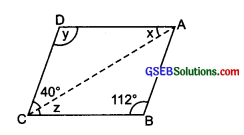y = 112°
In ∆ ACD, x + y + 40° = 180°
x + 112° + 40° = 180°
∴ x = 180° – 112° – 40° = 28°
∵ AD || BC and AC is a transversal.
∴ x = z [∵ Alternate angles are equal]
and z = 28°
Thus, x = 28°, y = 112° and z = 28°Question 3.
Can a quadrilateral ABCD be a parallelogram if
(i) ∠D + ∠B = 180°?
(ii) AB = DC = 8 cm, AD = 4 cm and BC = 4.4 cm?
(in) ∠A = 70° and ∠C = 65°?
Solution:
∠A + ∠B = Sum of adjacent angles = 180°
∴ The quadrilateral may be a parallelogram but not always

AB = DC = 8 cm
BC = 4.4 cm
∵ Opposite sides AD and BC are not equal.
∴ It cannot be a parallelogram.∠A = 70° and ZC = 65°
∵ Opposite angles ∠A ≠ ∠C
∴ It cannot be a parallelogram.

Question 4.
Draw a rough figure of a quadrilateral that is not a parallelogram but has exactly two opposite angles of equal measure.
Solution:
In the adjoining figure, ABCD is not a parallelogram such that opposite angles ∠B and ∠D are equal. It is a kite.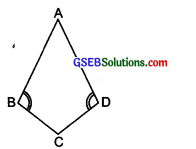Question 5.
The measures of two adjacent angles of a parallelogram are in the ratio 3 : 2. Find the measure of each of the angles of the parallelogram.
Solution:
Let ABCD be a parallelogram in which adjacent angles ∠A and ∠B are 3x and 2x respectively, since adjacent angles are supplementary.
∴ ∠A + ∠B = 180°
∴ 3x + 2x = 180° or 5x = 180°
or x = $$\frac { 180° }{ 5 }$$ = 36°
∠A = 3 x 36° = 108° and ∠B = 2 x 36° = 72°
∵ Opposite angles are equal.
∴∠D = ∠B = 72° and ∠C = ∠A = 108°
∴ ∠A = 108°, ∠B = 12°, ∠C = 108° and ∠D = 72°Question 6.
Two adjacent angles of a parallelogram have equal measure. Find the measure of each of the angles of the parallelogram.
Solution:
Let ABCD be a parallelogram such that adjacent angles ∠A = ∠B.
Since ∠A + ∠B = 180°
∠A = ∠B = $$\frac { 180° }{ 2 }$$ = 90°
Since, opposite angles of a parallelogram are equal.
∠A = ∠C = 90° and ∠B = ∠D = 90°
Thus, ∠A = 90°, ∠B = 90°, ∠C = 90° and ∠D = 90°.

Question 7.
The adjacent figure HOPE is a parallelogram. Find the angle measures x, y and z. State the properties you use to find them.
Solution: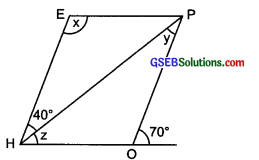y + z = 70° …..(1)
In a triangle, exterior angle is equal to the sum of opposite interior angles.
∴ In A ∠HOP, ∠HOP = 180° – (y + z)
= 180° – 70° = 110°
Now x = ∠HOP
[Opposite angles of a parallelogram are equal]
∴ x = 110°
EH || OP and PH is a transversal.
∴ y = 40° [Alternate angles are equal]
From (1), 40° + z = 70°
∴ z = 70° – 40° = 30°
Thus, x = 110°, y = 40° and z = 30°Question 8.
The following figures GUNS and RUNS are parallelograms. Find x and y. (Lengths are in cm.)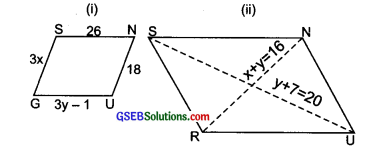(i) ∵ GUNS is a parallelogram.
Its opposite sides are equal.
GS = NU and SN = GU
or 3x = 18 and 26 = 3y – 1
Now 3x = 18 ⇒ x = $$\frac { 18 }{ 3 }$$ = 6
3y – 1 = 26 ⇒ y = $$\frac { 26 + 1 }{ 3 }$$ = $$\frac { 27 }{ 3 }$$ = 9
Thus, x = 6 cm and y = 9 cm

(ii) RUNS is a parallelogram and thus its diagonals bisect each other.
∴ x + y = 16
and 7 + y = 20
i.e y = 20 – 7
or y = 13
∴ From x + y = 16, we have
x + 13 = 16
or x = 16 – 13 = 3
Thus, x = 3 cm and y = 13 cm

Question 9.
In the following figure, both RISK and CLUE are parallelograms. Find the value of x.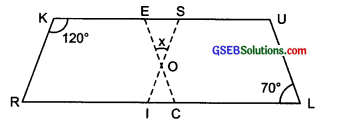Solution:
RISK is a parallelogram.
∴ ∠R + ∠K= 180°
[∵ Adjacent angles of a parallelogram are supplementary]
or ∠R + 120° = 180°
⇒ ∠R = 180° – 120° = 60°
But ∠R and ∠S are opposite angles.
∴ ∠S = 60°
CLUE is also a parallelogram.
∴ Its opposite angles are equal.
∴ ∠E = ∠L = 70°
Now, in ∆ ESO, we have
∠E + ∠S + x = 180°
∴ 70° + 60° + x = 180°
or x = 180° – 60° – 70° ⇒ x = 50°

Question 10.
Explain how this figure is a trapezium. Which of its two sides are parallel?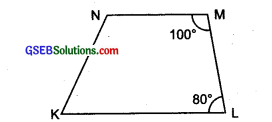Solution:
Since, 100° + 80° = 180°
i.e., ∠M and ∠L are supplementary.
NM || KL
[∵ If interior opposite angles along the transversal are supplementary]

Question 11.
Find m∠C in the adjoining figure if $$\bar { AB }$$ || $$\bar { DC }$$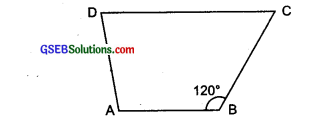Solution:
∵ ABCD is a trapezium in which $$\bar { AB }$$  || $$\bar { DC }$$ and BC is a transversal.
∵ Interior opposite angles along BC are supplementary.
∴ m∠B + m∠C = 180° or m∠C = 180° – m∠B
∴ m∠C = 180° – 120° [∴∠B = 120°]
or m∠C = 60°Question 12.
Find the measure of ∠P and ∠S if $$\bar { SP }$$ || $$\bar { RQ }$$ in figure. (If you find m∠R, is there more than one method to find m∠P?)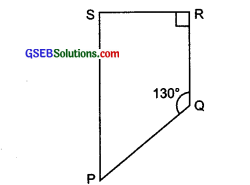Solution:
PQRS is a trapezium such that $$\bar { SP }$$ || $$\bar { RQ }$$ and PQ is a transversal
∴ m∠p + m∠Q = 180°
[Interior opposite angles are supplementary]
or m∠p + 130° = 180°
or m∠P = 180° – 130° = 50°
Again SP || RQ and RS is a transversal
∴ m∠S + m∠R = 180°
or m∠S + 90° = 180°
∴ m∠S = 108° – 90° = 90°
Yes, using the angle sum property of a quadrilateral, we can find m∠p when m∠R is know.
∴ m∠P +m∠Q + m∠R + m∠S = 360°
or m∠P + 130° + 90° + 90° = 360°
or m∠P = 360° – 130° – 90° – 90° = 50°